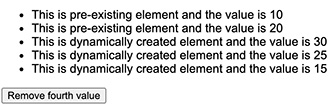# D3.js 数据连接 Data Join

## 数据连接如何工作？

``````[10, 20, 30, 25, 15]
``````

`HTML`

``````<ul id = "list">
<li><li>
<li></li>
</ul>
``````

### D3.js 代码

``````d3.select("#list").selectAll("li").data([10, 20, 30, 25, 15]);
``````

``````1. li - 10
2. li - 20
``````

``````d3.select("#list").selectAll("li")
.data([10, 20, 30, 25, 15])
.text(function(d) { return d; });
``````

text() 方法中的函数用于获取 li 元素映射数据。这里，`d` 代表第一个 li 元素的10和第二个 li 元素的20 。

``````3. li - 30
4. li - 25
5. li - 15
``````

``````d3.select("#list").selectAll("li")
.data([10, 20, 30, 25, 15])
.text(function(d) { return "This is pre-existing element and the value is " + d; })
.enter()
.append("li")
.text(function(d)
{ return "This is dynamically created element and the value is " + d; });
``````

``````d3.selectAll("li")
.data([10, 20, 30, 15])
.exit()
.remove()
``````

``````<!DOCTYPE html>
<html>
<script type = "text/javascript" src = "https://d3js.org/d3.v6.min.js"></script>
<style>
body { font-family: Arial; }
</style>

<body>
<ul id = "list">
<li></li>
<li></li>
</ul>

<input type = "button" name = "remove" value = "Remove fourth value"
onclick = "javascript:remove()" />

<script>
d3.select("#list").selectAll("li")
.data([10, 20, 30, 25, 15])
.text(function(d)
{ return "This is pre-existing element and the value is " + d; })
.enter()
.append("li")
.text(function(d)
{ return "This is dynamically created element and the value is " + d; });

function remove() {
d3.selectAll("li")
.data([10, 20, 30, 15])
.exit()
.remove()
}
</script>
</body>
</html>
``````